Next: The equilibrium period Up: Evolution of Magnetic Rotators Previous: Evolution of Magnetic Rotators

### The evolution equation

Analysis of the nature of interaction of a magnetized star with the surrounding plasma allows us to write an approximate evolution equation for the angular momentum of a magnetic rotator in the general form (Lipunov, 1982a)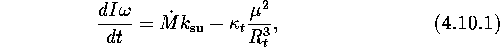whereis a specific angular momentum applied by the accretion matter to the rotator. This quantity is given by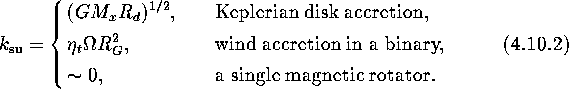where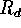is the radius of the inner disk edge,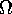is the rotational frequency of the binary system, and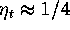(Illarionov and Sunyaev, 1975). The values of dimensionless factor, characteristic radius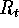and the accretion ratein different regimes are presented in Table 3.

The evolution equation (4.10.2) is approximate. In practice, the situation with propellers  and superpropellers  is not yet clear. In Table 3is the size of a magnetosphere  whose value at the propeller stage is not known accurately and which may differ significantly from the standard expressions for the Alfvén radius.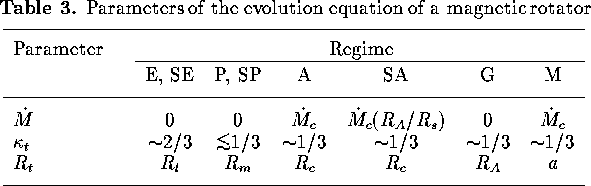Mike E. Prokhorov
Sat Feb 22 18:38:13 MSK 1997